barplot(GNP ~ Year, data = longley)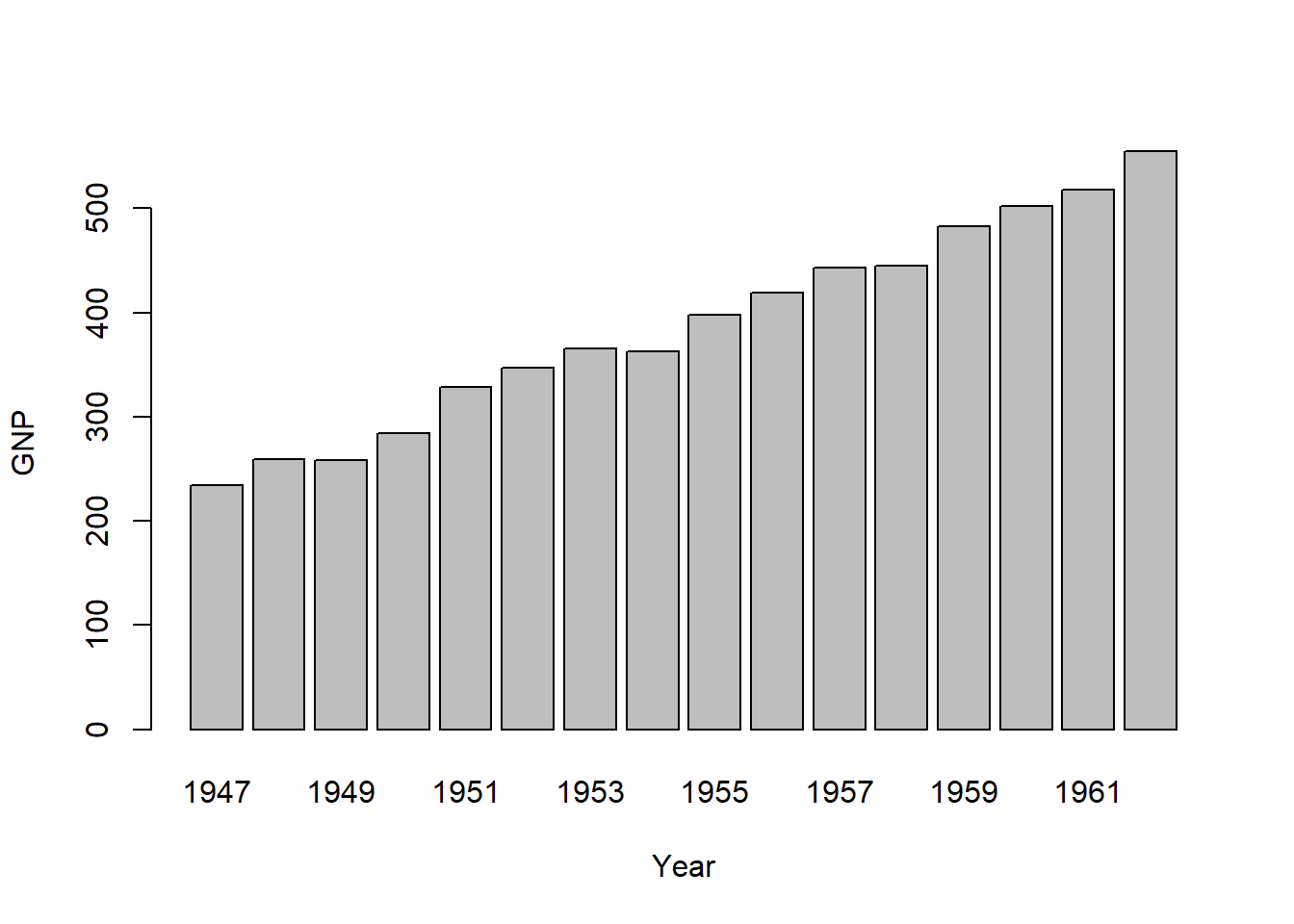barplot(cbind(Employed, Unemployed) ~ Year, data = longley)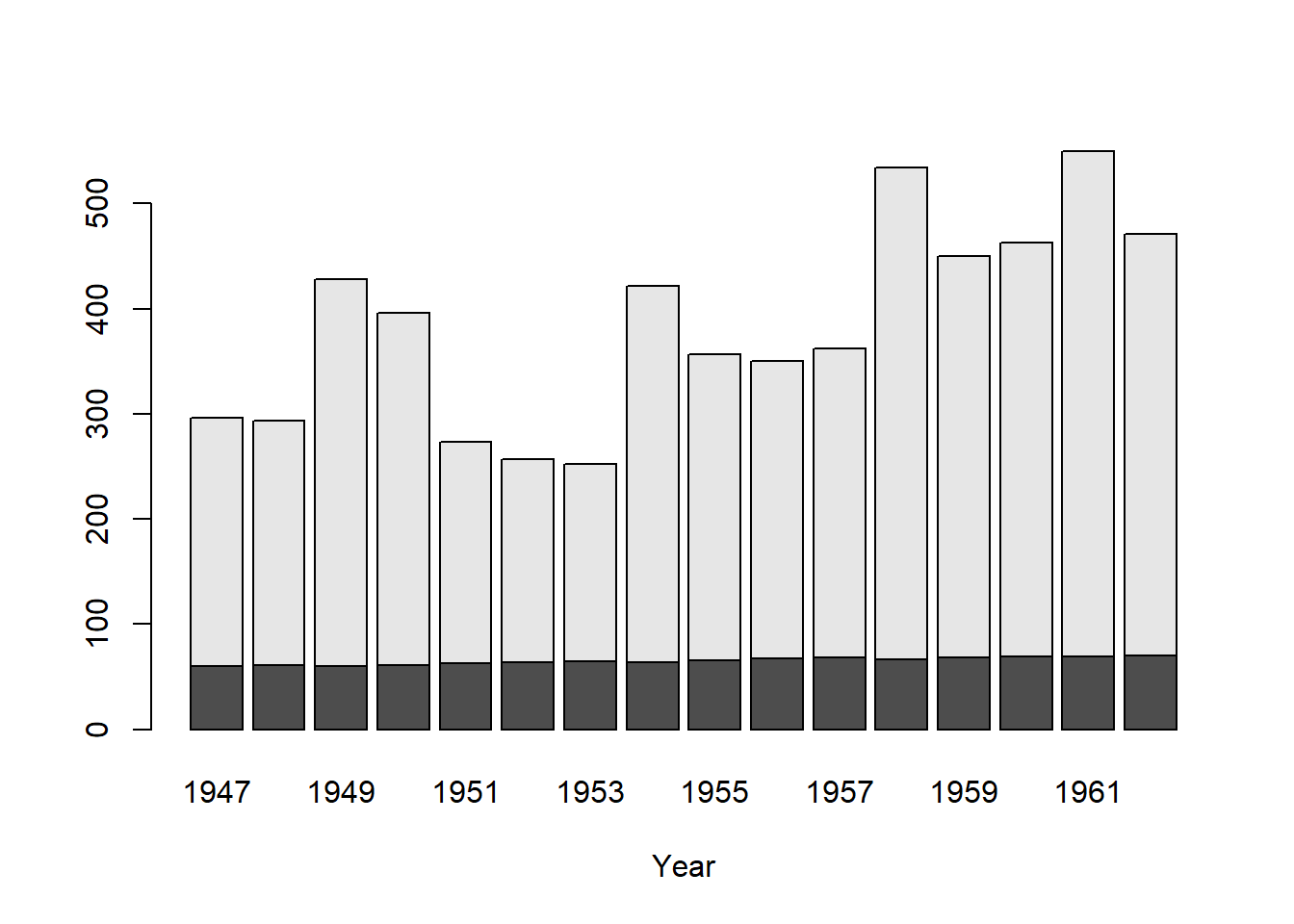op <- par(mfrow = 2:1, mgp = c(3,1,0)/2, mar = .1+c(3,3:1))
summary(d.Titanic <- as.data.frame(Titanic))
##   Class       Sex        Age     Survived      Freq
##  1st :8   Male  :16   Child:16   No :16   Min.   :  0.00
##  2nd :8   Female:16   Adult:16   Yes:16   1st Qu.:  0.75
##  3rd :8                                   Median : 13.50
##  Crew:8                                   Mean   : 68.78
##                                           3rd Qu.: 77.00
##                                           Max.   :670.00
barplot(Freq ~ Class + Survived, data = d.Titanic,
subset = Age == "Adult" & Sex == "Male",
main = "barplot(Freq ~ Class + Survived, *)", ylab = "# {passengers}", legend = TRUE)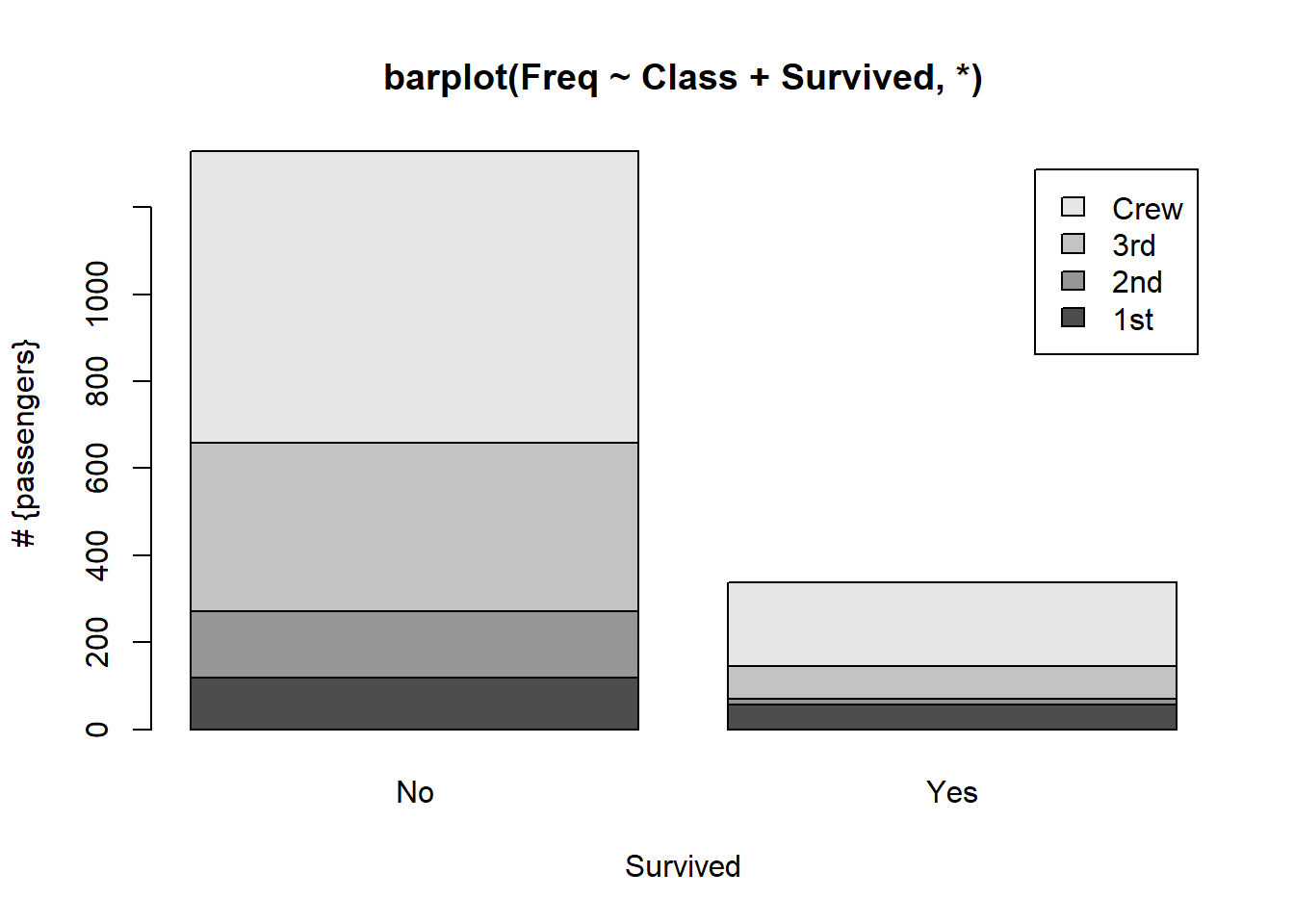# Corresponding table :
(xt <- xtabs(Freq ~ Survived + Class + Sex, d.Titanic, subset = Age=="Adult"))
## , , Sex = Male
##
##         Class
## Survived 1st 2nd 3rd Crew
##      No  118 154 387  670
##      Yes  57  14  75  192
##
## , , Sex = Female
##
##         Class
## Survived 1st 2nd 3rd Crew
##      No    4  13  89    3
##      Yes 140  80  76   20
# Alternatively, a mosaic plot :
mosaicplot(xt[,,"Male"], main = "mosaicplot(Freq ~ Class + Survived, *)", color=TRUE)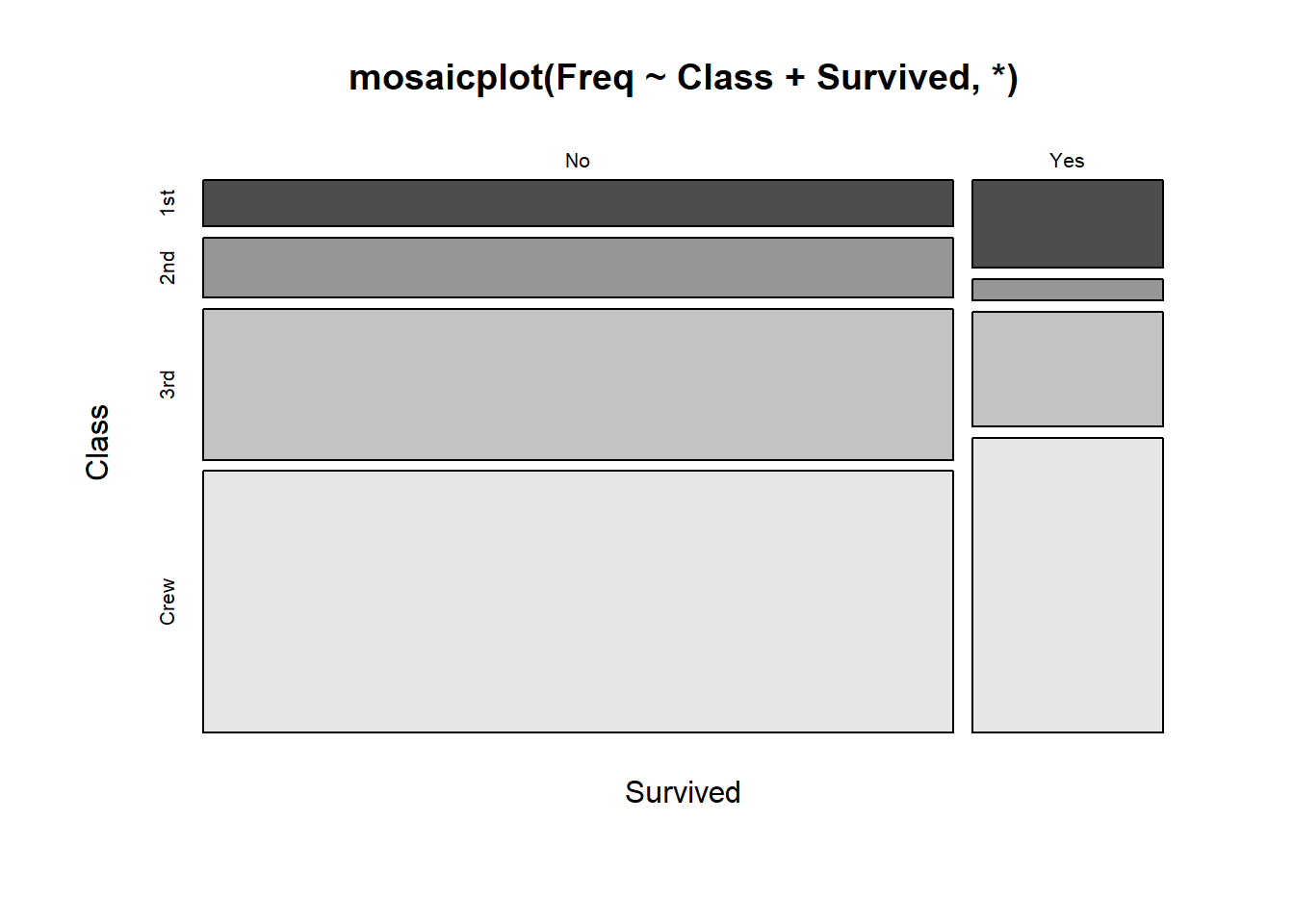par(op)
# Default method
require(grDevices) # for colours
tN <- table(Ni <- stats::rpois(100, lambda = 5))
r <- barplot(tN, col = rainbow(20))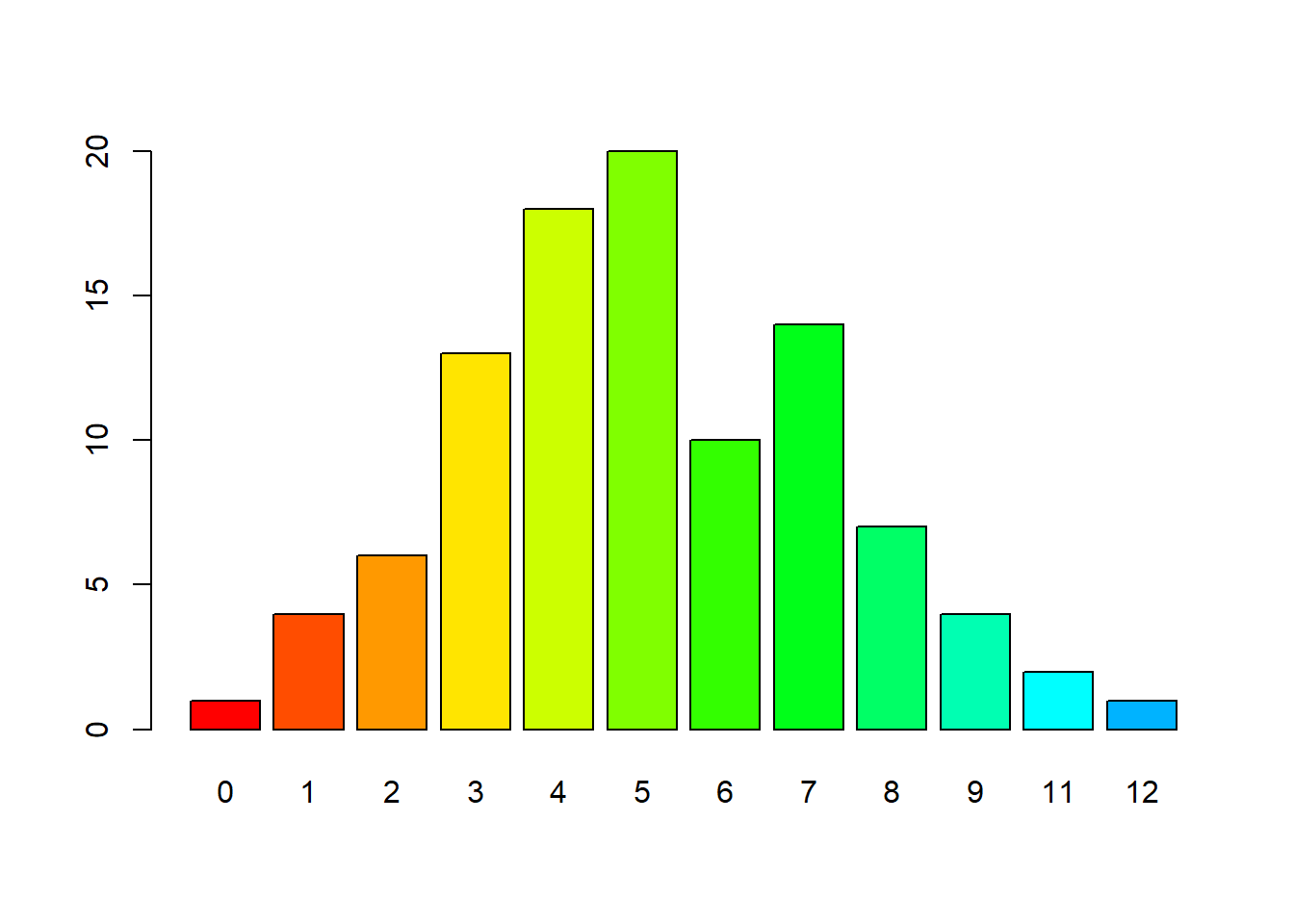barplot(tN, space = 1.5, axisnames = FALSE,
sub = "barplot(..., space= 1.5, axisnames = FALSE)")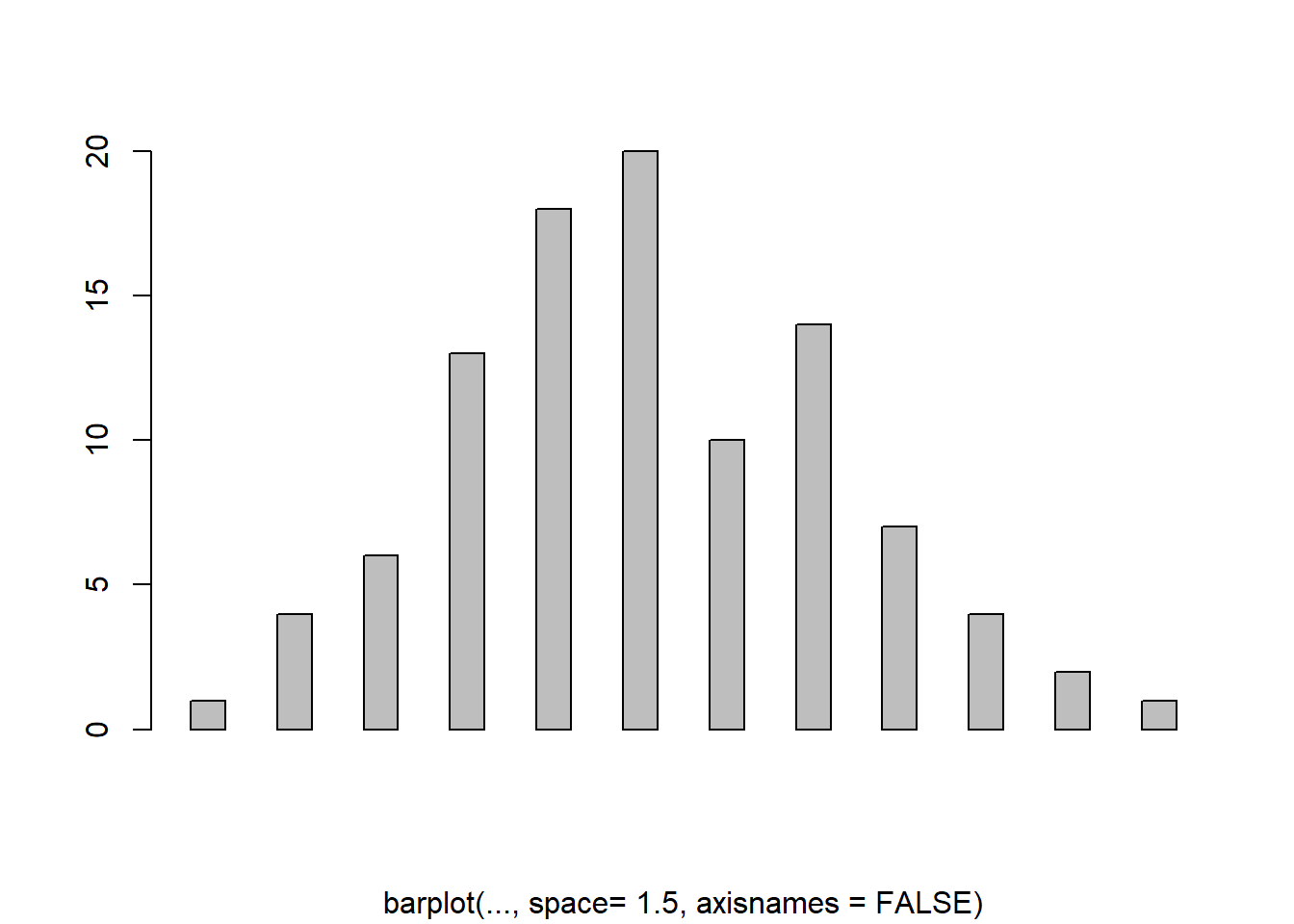barplot(VADeaths, plot = FALSE)
##  0.7 1.9 3.1 4.3
barplot(VADeaths, plot = FALSE, beside = TRUE)
##      [,1] [,2] [,3] [,4]
## [1,]  1.5  7.5 13.5 19.5
## [2,]  2.5  8.5 14.5 20.5
## [3,]  3.5  9.5 15.5 21.5
## [4,]  4.5 10.5 16.5 22.5
## [5,]  5.5 11.5 17.5 23.5
mp <- barplot(VADeaths) # default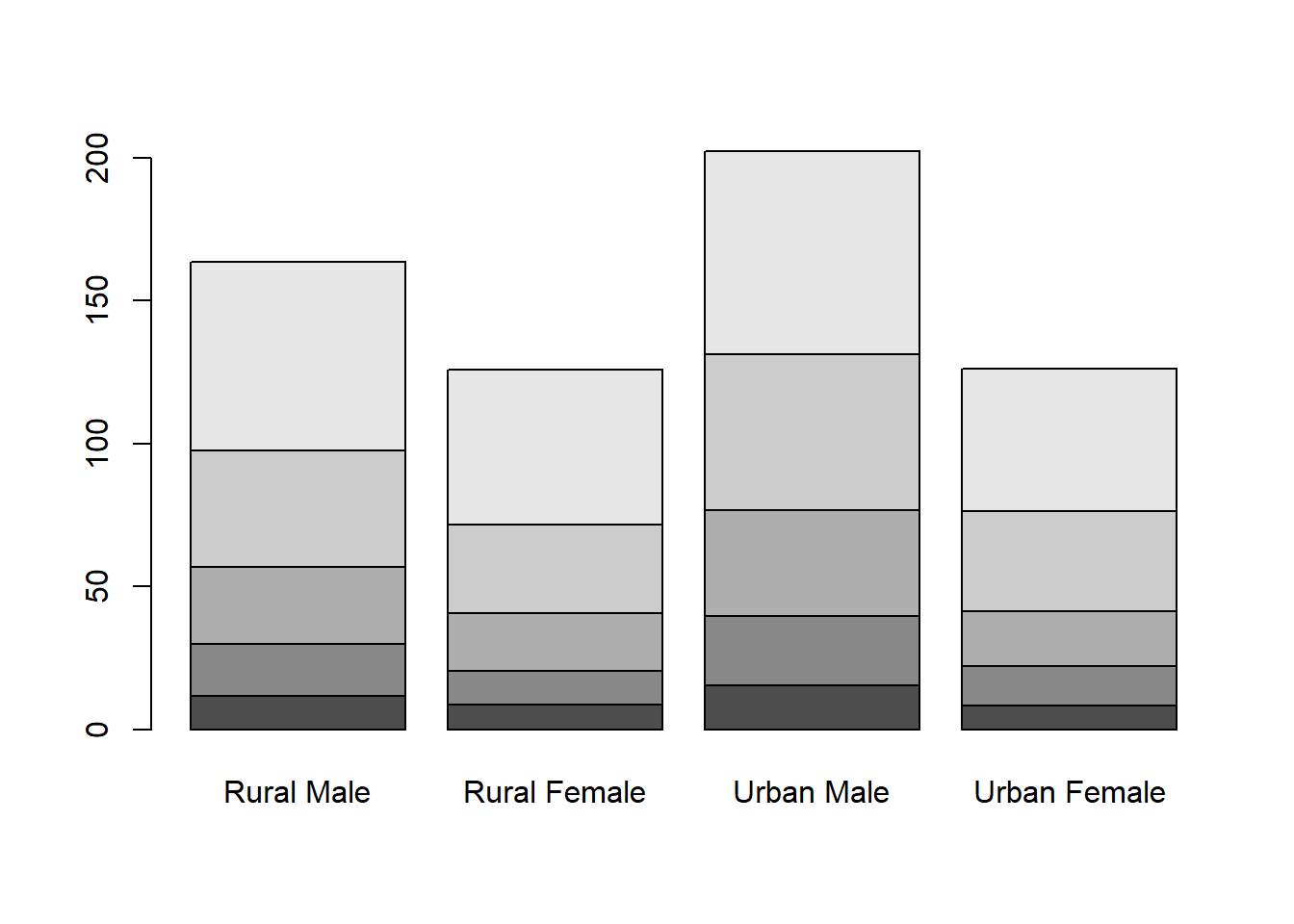mp <- barplot(VADeaths) # default
text(mp, tot + 3, format(tot), xpd = TRUE, col = "blue")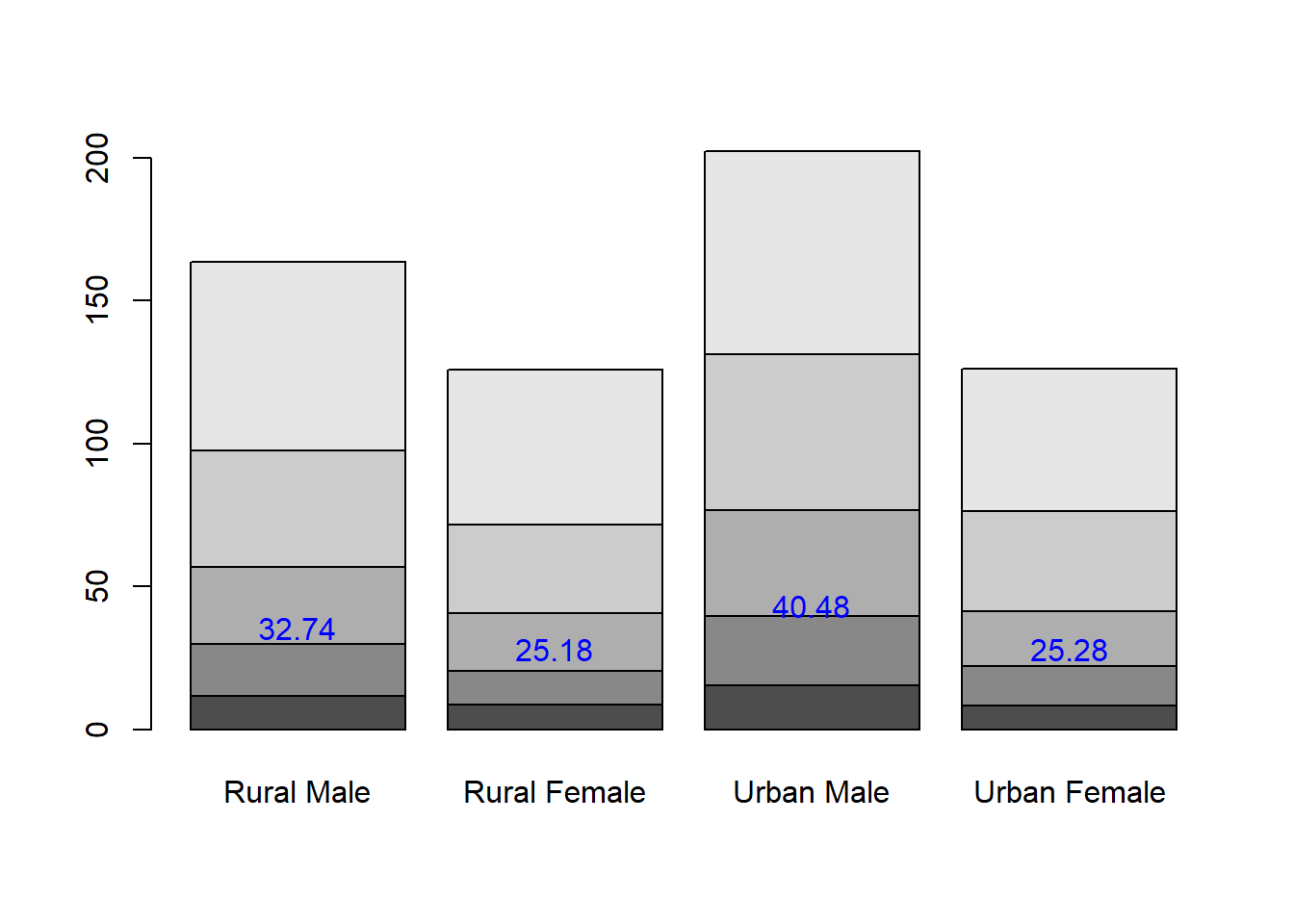barplot(VADeaths, beside = TRUE,
col = c("lightblue", "mistyrose", "lightcyan",
"lavender", "cornsilk"),
legend = rownames(VADeaths), ylim = c(0, 100))
title(main = "Death Rates in Virginia", font.main = 4)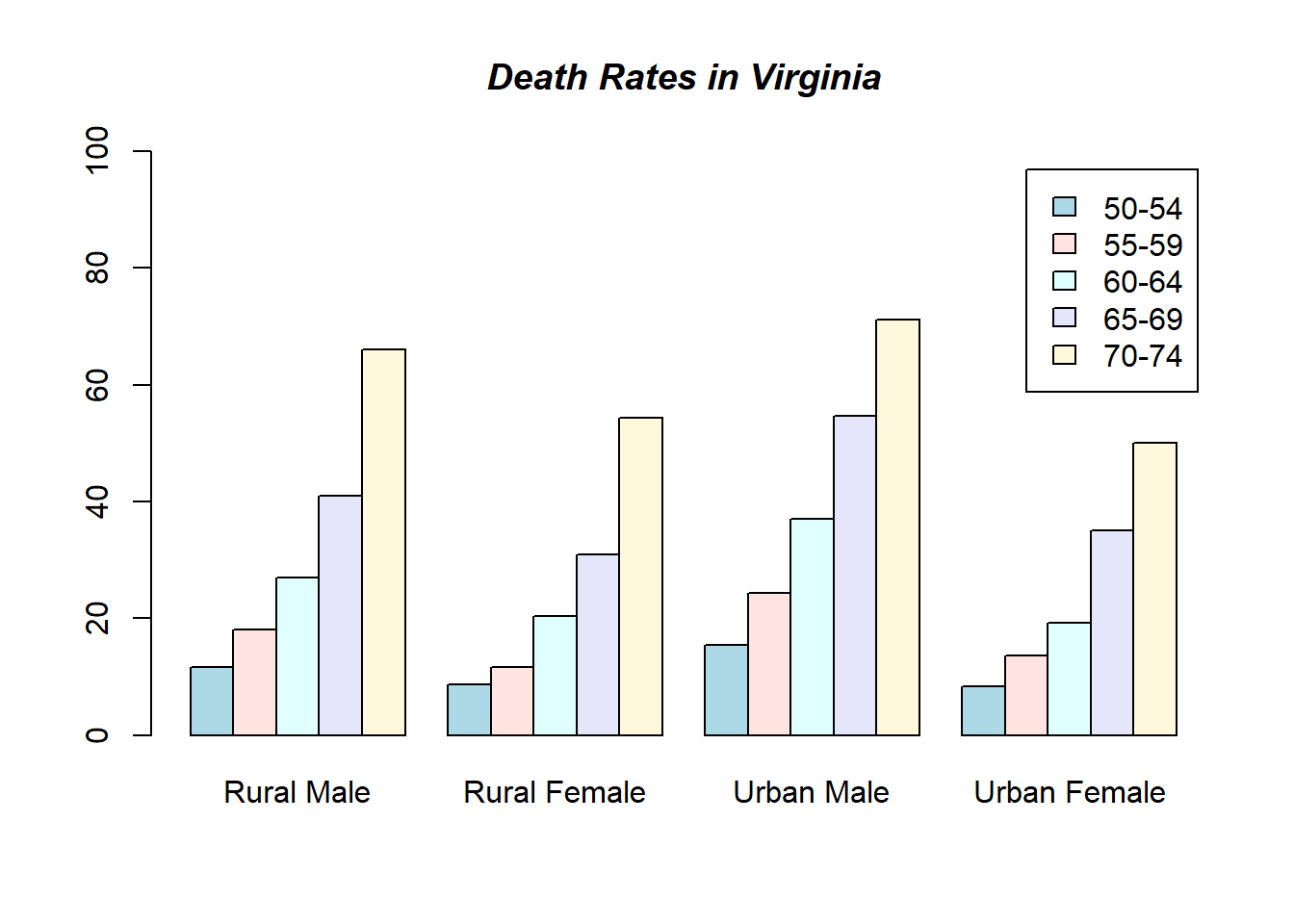hh <- t(VADeaths)[, 5:1]
mybarcol <- "gray20"
mp <- barplot(hh, beside = TRUE,
col = c("lightblue", "mistyrose",
"lightcyan", "lavender"),
legend = colnames(VADeaths), ylim = c(0,100),
main = "Death Rates in Virginia", font.main = 4,
sub = "Faked upper 2*sigma error bars", col.sub = mybarcol,
cex.names = 1.5)
segments(mp, hh, mp, hh + 2*sqrt(1000*hh/100), col = mybarcol, lwd = 1.5)
stopifnot(dim(mp) == dim(hh))  # corresponding matrices
mtext(side = 1, at = colMeans(mp), line = -2,
text = paste("Mean", formatC(colMeans(hh))), col = "red")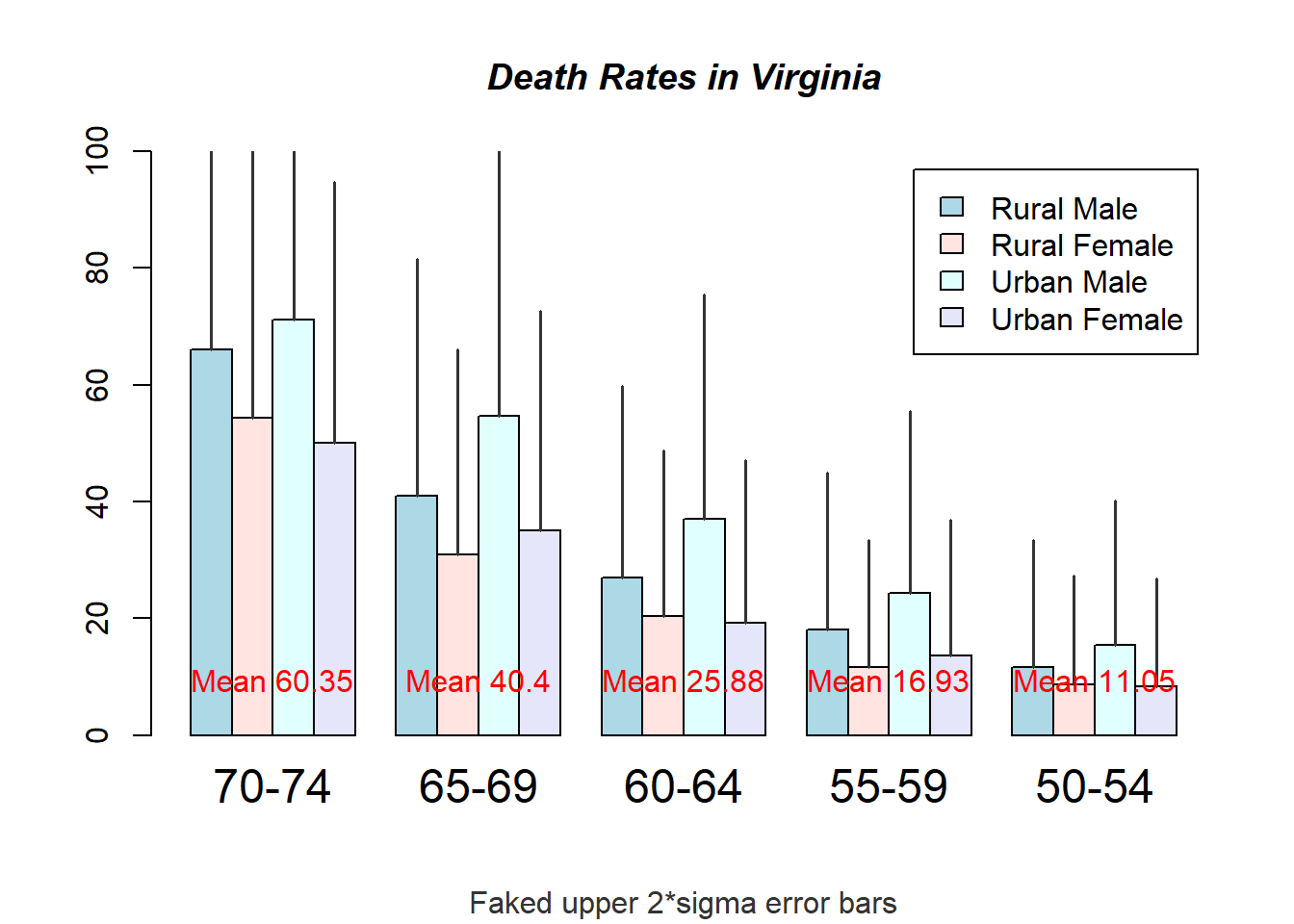# Bar shading example
barplot(VADeaths, angle = 15+10*1:5, density = 20, col = "black",
title(main = list("Death Rates in Virginia", font = 4))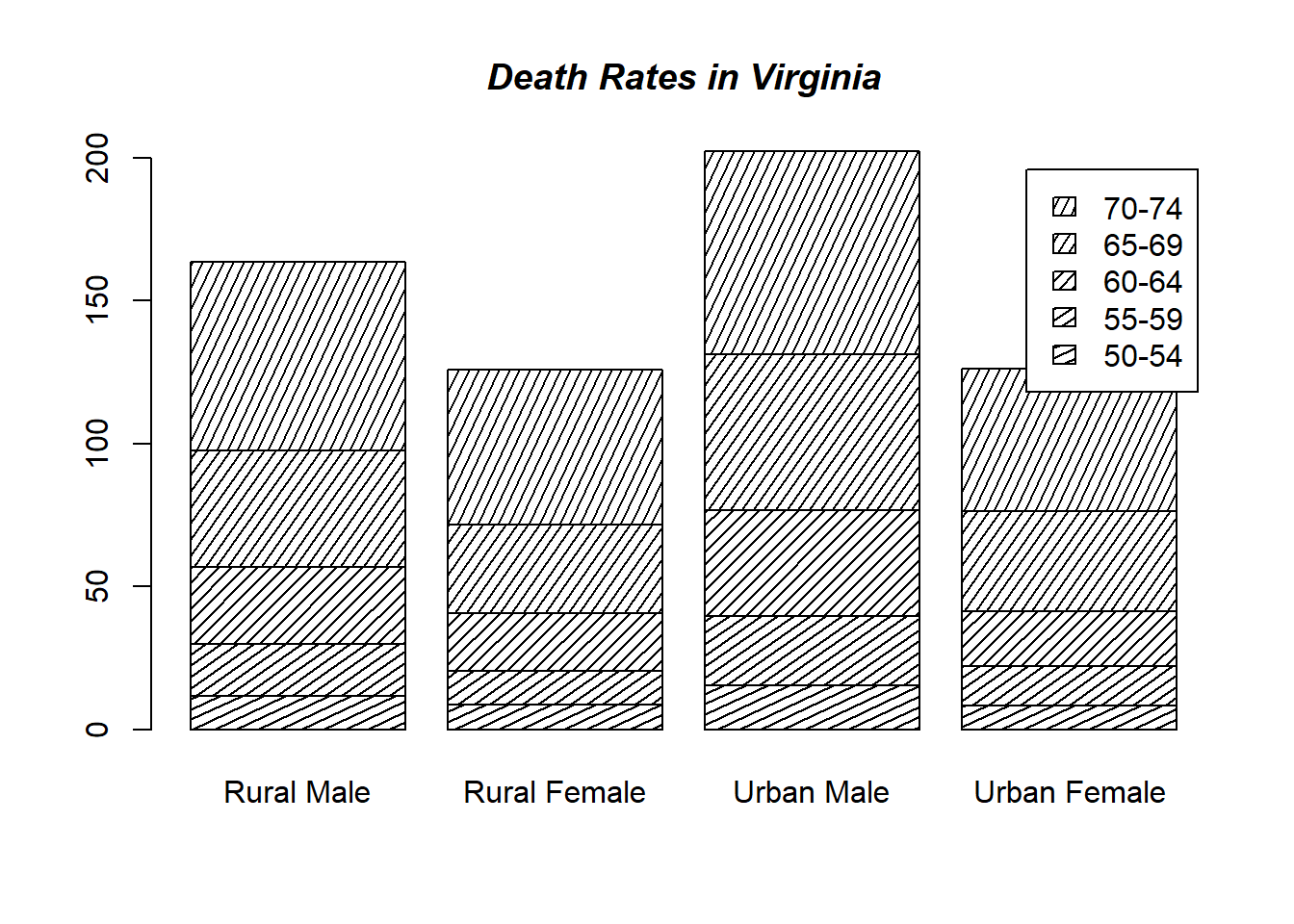# Border color
barplot(VADeaths, border = "dark blue")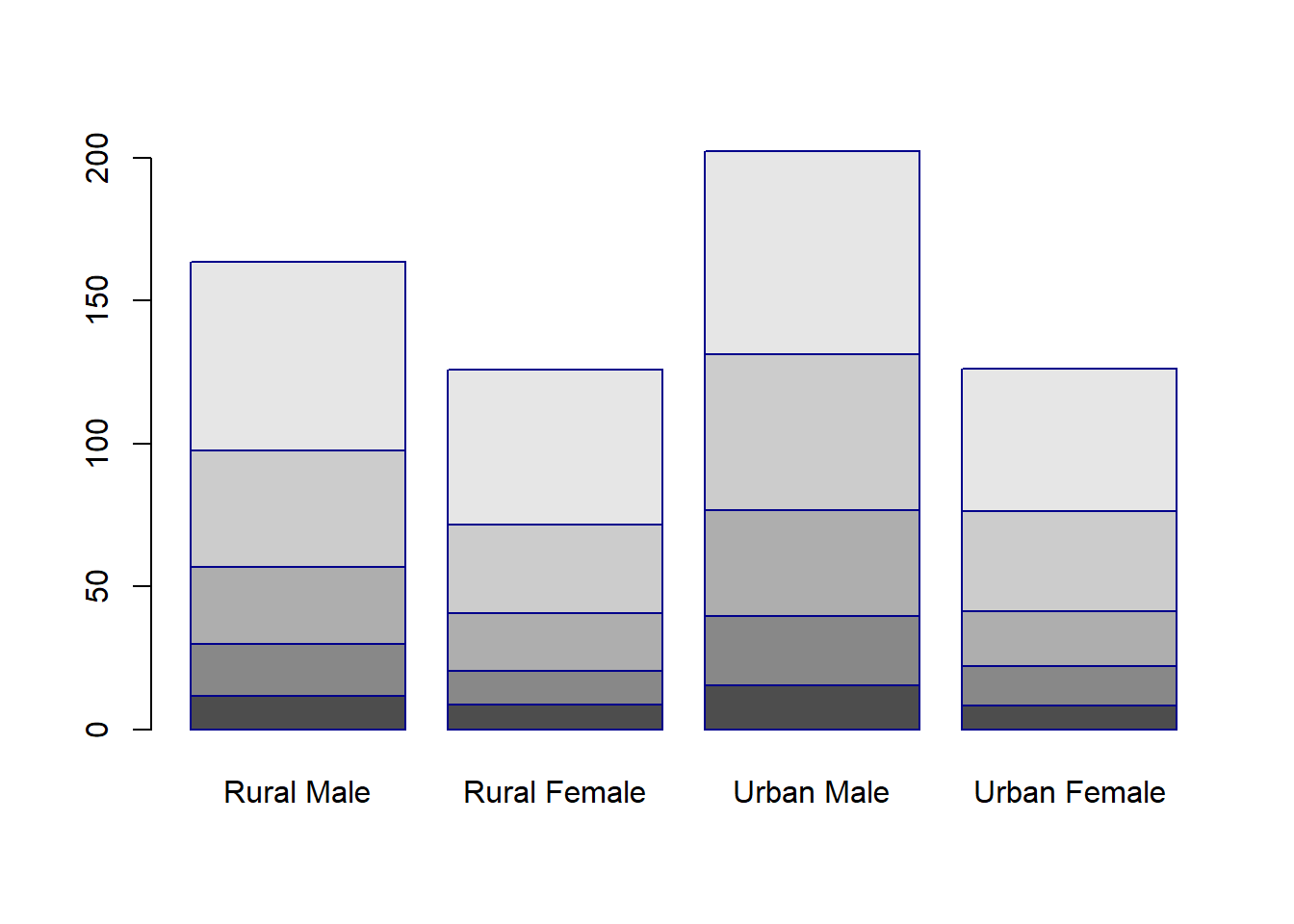# Log scales (not much sense here)
barplot(tN, col = heat.colors(12), log = "y")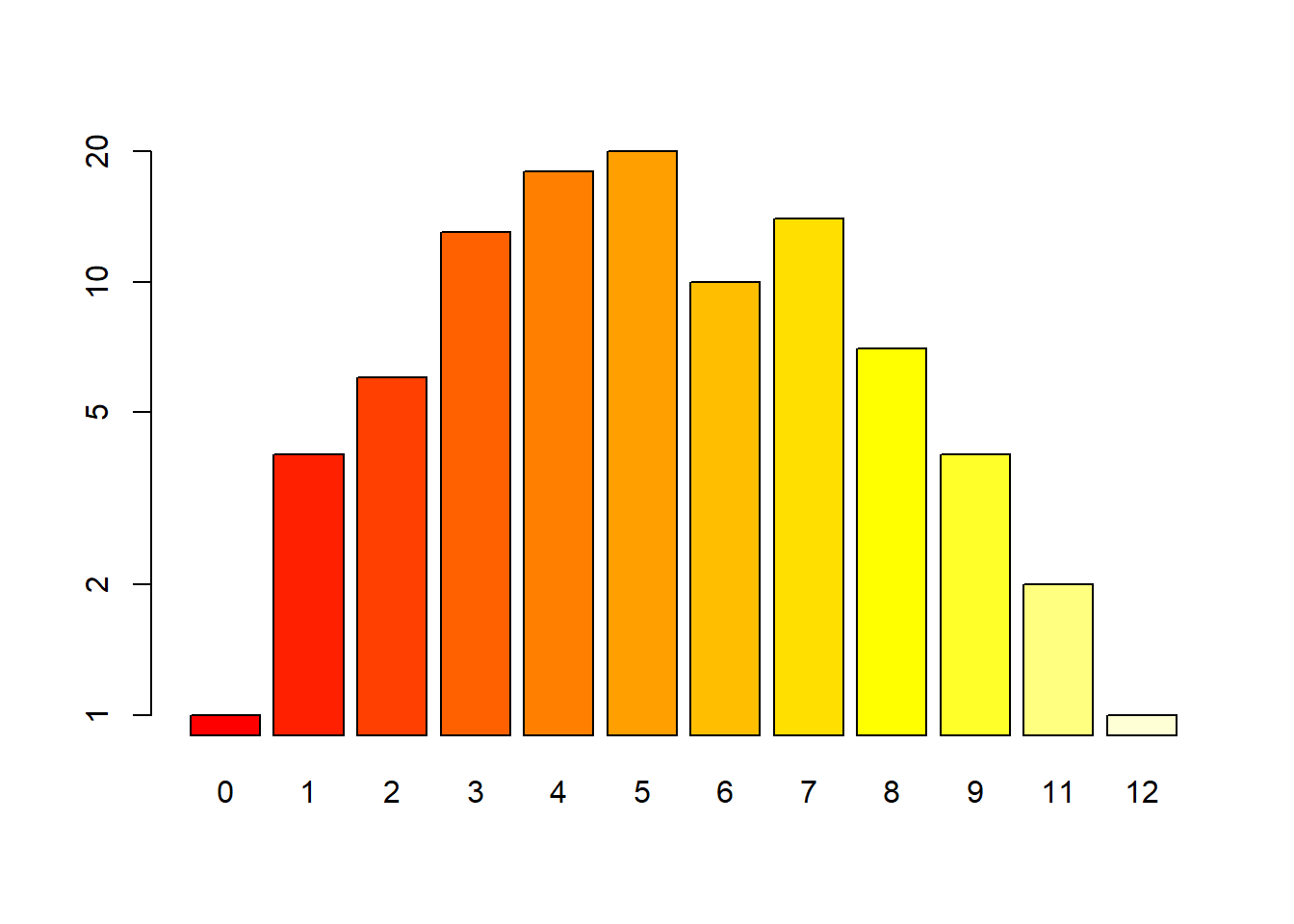barplot(tN, col = gray.colors(20), log = "xy")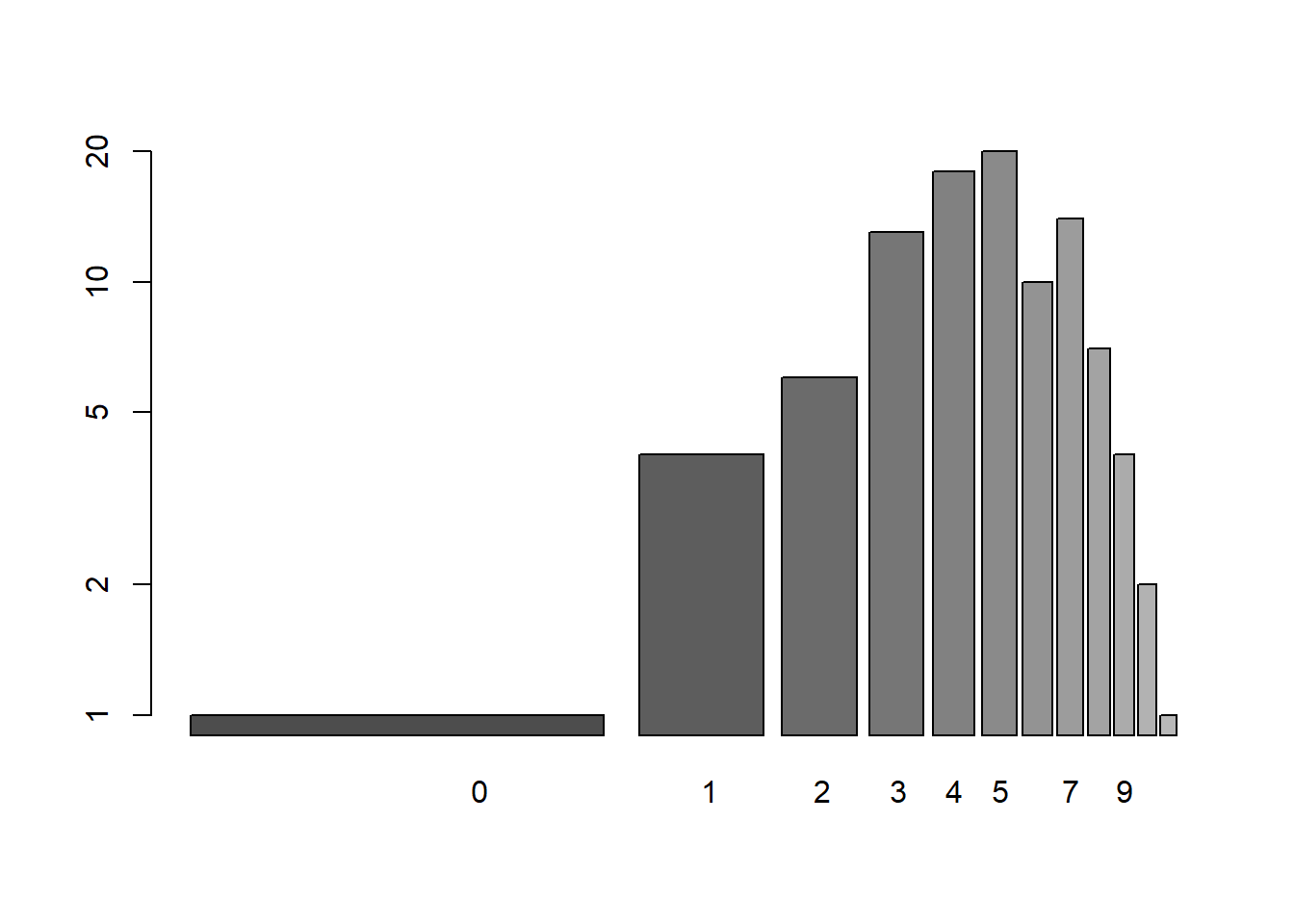# Legend location
barplot(height = cbind(x = c(465, 91) / 465 * 100,
y = c(840, 200) / 840 * 100,
z = c(37, 17) / 37 * 100),
beside = FALSE,
width = c(465, 840, 37),
col = c(1, 2),
legend.text = c("A", "B"),
args.legend = list(x = "topleft"))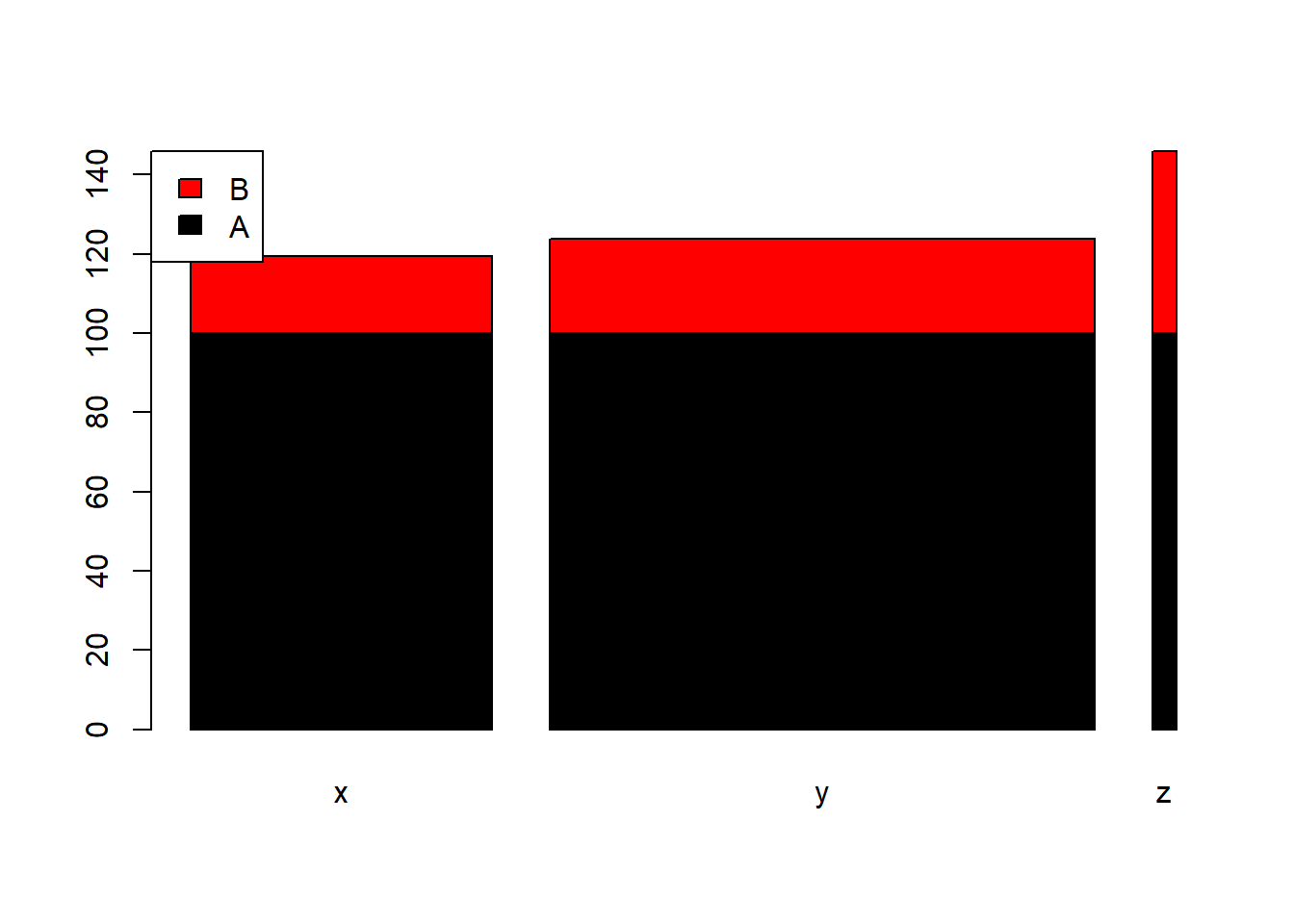# }